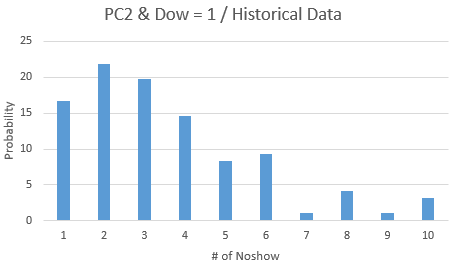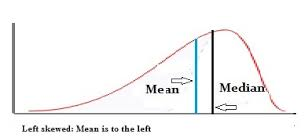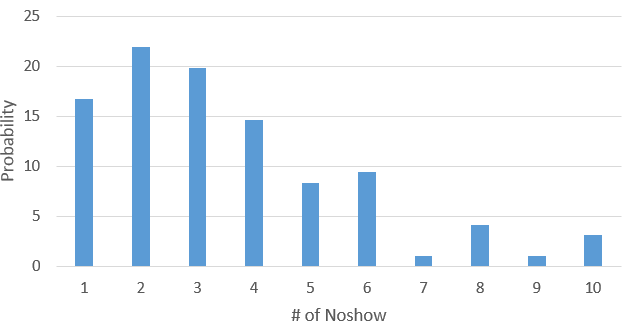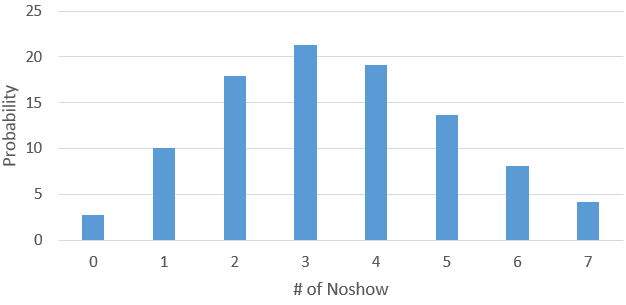## According to a group of data, how to simulate a distribution

Hi, I have a group of data as following: Value / Count (Frequency) / Probability among all:

And I make a histogram on Probability as attachemnt. While I would like to make the percentage increasing before it reaches mean; and decreasing after it reaches mean. How could I change the data to satisfy that? (Like the trend of Poisson or Normal Distribution)

And can it be a procedure that automatically do that?

Thank you!

Value        Count      Probability

 1 16 16.6667 2 21 21.875 3 19 19.7917 4 14 14.5833 5 8 8.33333 6 9 9.375 7 1 1.04167 8 4 4.16667 9 1 1.04167 10 3 3.12514 REPLIES 14

## Re: According to a group of data, how to simulate a distribution

I think you need to show an example of what you are looking for as output. I am not quite sure what you mean by  percentage increasing before it reaches mean. How is the posted data going to be related to the output?

## Re: According to a group of data, how to simulate a distribution

Hi,

Sorry for not explaining well. I would like the output to be like normal or poisson distribution or left skewed is okay. Like the one in the attachment. So that I may need to change the data value. Thanks!Ksharp
Super User

## Re: According to a group of data, how to simulate a distribution

So you want bell shape (normal) distribution?

better post it at IML forum.

Check PROC MCMC to us Box-Cox transformation to make it as normal distribution.

## Re: According to a group of data, how to simulate a distribution

Thank you!

I will check on it, And post at IML board if I still have no idea.Ksharp
Super User

## Re: According to a group of data, how to simulate a distribution

```
Maybe you want this.

http://blogs.sas.com/content/iml/2016/11/02/reverse-data-before-fit-distribution.html

```

## Re: According to a group of data, how to simulate a distribution

Hi Ksharp,

Sorry for my late response.

The initial probability table looks like this:For example, the above figure. I want the Probability of 'No-show' = 6 be smaller than 'No-show' = 5; and 'No-show' = 7 be in the middle of 'No-show' = 6 & 9. Which looks like a Normal or Poisson Distribution, and finally returns probability for each value of 'No-show'.

My current approach is to obtain mean for this group, and generate Poisson Distribution according to it.

Like the following (Not exact the same data, but similar case)In this way, the probability keeps increasing before the mean value, and then keeps decreasing after that.

Is there any other approach? Thank you!

## Re: According to a group of data, how to simulate a distribution

If you have the original data, you can use a bootstrap (or smoothed bootstrap) technique to simulate the data.

If you only have the quantiles, you can simulate the (approximate) distribution from a piecewise linear approximation to the empirical CDF.  The technique uses the inverse CDF method to simulate from the approximate empirical CDF.

## Re: According to a group of data, how to simulate a distribution

Hi Rick,

For this particular question,

My initial probability distributon is like this :This is the actrual case, however, I want to adust the probability ofr some values: For example, in the above figure. I want the Probability of 'No-show' = 6 be smaller than 'No-show' = 5; and 'No-show' = 7 be in the middle of 'No-show' = 6 & 9. Which looks like a Normal or Poisson Distribution, and finally returns probability for each value of 'No-show'.

My current approach is to obtain mean for this group, and generate Poisson Distribution according to it.

Like the following (Not exact the same data, but similar case) :So that in this way, the probability is increasing before the mean and then decreasing after it.

Is there any other approach? Thank you!

## Re: According to a group of data, how to simulate a distribution

It sounds like you are trying to transform the distribution towards normality. Usually, you would use a LOG transformation or a square-root transformation to transform a continuous distribution into another continuous distribution, but you seem to want to transform a discrete distribution. It is not clear to me if the new distribution is supposed to be discrete or continuous.  If it can be continuous, try the LOG transformation on your data.

I think it would be helpful if you describe what you are trying to accomplish scientifically. What are the data? What scientific question are you trying to answer or model?  Why are you trying to transform the data towards normality?

## Re: According to a group of data, how to simulate a distribution

Hi Rick,

The data is historical 'No-show' information. ('No-show' mean already booked but not appear in hospitality industry) so that it must be integer (not continuous).

And X-axis is the count for 'No-show' that appears in the past: Y-axis is its regarding probability. ('No-show' = 1 takes about 17% and 'No-show' = 2 takes about 22%).

In real case, probability for 'No-show' = 6 might be higher than 'No-show' = 5 and also higher than 'No-show' = 7. However, I would like to transfer the data so that the probability for 'No-show' = 6 is between 'No-show' = 5 and 'No-show' = 7. Normality will work I think, but data should be discrete instead of continuous (that's why I tried Poisson myself). In doing so, it would be easier to interpret the distribution to the business.

Thnak you!

## Re: According to a group of data, how to simulate a distribution

> In real case, probability for 'No-show' = 6 might be higher than 'No-show' = 5 and also higher than 'No-show' = 7.

Not sure what "in real case" means. You say this is historical data, so the data is real, right?

Are either of the following what you are trying to do?

1. Adjust/modify the observed proportions so that they better fit some theoretical model or pre-held beliefs.

2. Find some discrete parametric distribution that you can fit to the data. You want the fitted distribution to look somewhat normal.

## Re: According to a group of data, how to simulate a distribution

Hi Rick,

Thanks! And in real case means historical data, and it is real as you said.

And the second thing you mentioned is that I want, which is to fit the distribution to look somewhat normal.

## Re: According to a group of data, how to simulate a distribution

You can't change the distribution. The data have the shape that they have. What you can do is fit a discrete distribution to the data. I still don't understand how the data are generated, so I can't recommend whether you should use a Poisson, binomial, or something else. However, there is a SAS Knowledge Base article that shows how to fit discrete distributions:

http://support.sas.com/kb/48/914.html

http://support.sas.com/kb/24/166.html

Here's a fit to the binomial distribution:

``````data A;
input freq @@;
N = _N_;
NTrials = 96;    /* you need the sample size to model the Binomial distrib */
datalines;
16 21 19 14 8 9 1 4 1 3
;
run;
proc means sum; run;

proc genmod data=A;
freq freq;
model N/NTrials = / dist=binomial;
output out=predbin p=p;
run;

proc print data=predbin(obs=1);
var p;   /* print the parameter estimate */
run;

data fit;
p = 0.037218;
set A;
pct = 100 * pdf("Binomial", N, p, NTrials);  /* expected values */
run;

proc sgplot data=fit;
vbar N / response=freq;         /* raw data */
vline N / response=pct markers; /* expected values under binomial model */
yaxis grid;
run;``````

## Re: According to a group of data, how to simulate a distribution

Hi Rick,

That's great. I will take a look at it and try.

Discussion stats
• 14 replies
• 1074 views
• 0 likes
• 4 in conversation